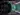# Quantum phase kickbackThe science of quantum computing is advancing quickly and has the potential to significantly alter the way we handle data. The notion of "quantum entanglement," in which two particles become inherently connected so that their characteristics become interdependent, is central to the field of quantum computing. This entanglement makes possible many other kinds of operations in quantum computing, including the one we'll be talking about here—the "phasekickback" operation.

In quantum computing, phasekickback is a method for controlling the phase of a quantum state. Knowing the meaning of "phase" in the context of quantum computing is crucial to understanding how it works.

The fundamental unit of information in quantum computing is called a qubit. A qubit, in contrast to a traditional bit, may be in more than one state at once; it can be both 0 and 1 at the same time. The phase of a qubit is the angle between the complex plane and the wave function.

A qubit's wave function may be represented as a vector in a Bloch sphere, a complex vector space with two dimensions. When this vector is projected onto the x-y plane, the angle between it and the x-axis equals the qubit's phase.

To achieve its results, phasekickback takes use of the entanglement between two qubits, A and B. Let's pretend qubit A is in a superposition of states, where it might be in either the 0 or 1 state with equal probability. Let's pretend for the moment that qubit B is in the 0 state. In this example, we'll use a controlled NOT (CNOT) gate to flip the state of qubit A if and only if qubit B is in the 1 state, where A is the control qubit and B is the target qubit.

This may seem to be a simple task, but the results are far from uninteresting. Now, by applying a phase gate (represented by U()) to qubit A, we can change the relative phase of the two superposition states in a way that depends on the state of qubit B. This phase shift is directly proportional to the dot product of the phase angle of the phase gate and the state of qubit B.

It is from this phenomenon that the name "phasekickback" originates. The phase shift on qubit A may be "kicked back" by manipulating the state of qubit B. A large variety of quantum computing operations may be accomplished with this very potent operation.

Phasekickback is utilised to implement some of the most important algorithms in quantum computing, such as the quantum Fourier transform (QFT). Cryptographically significant applications of the QFT include quantum algorithms like Shor's technique for factoring big integers.

The phase estimation technique, which finds the eigenvalues of a unitary operator, is only one example of a quantum algorithm that may be implemented using phasekickback.

Finally, phasekickback is an effective method in quantum computing for controlling the phase of a quantum state. Phasekickback is a technique for performing various quantum computing tasks, such as the execution of crucial quantum algorithms, by taking use of the entanglement of two qubits. It is anticipated that phasekickback will play an increasingly essential role in the promising area of quantum computing as it continues to grow.

XPERIMEN.COM